Saturday, July 11, 2020
Home > CBSE Class 12 > NCERT Solutions for Class 12 Maths Application of Integerals Miscellaneous Exercise

# NCERT Solutions for Class 12 Maths Application of Integerals Miscellaneous Exercise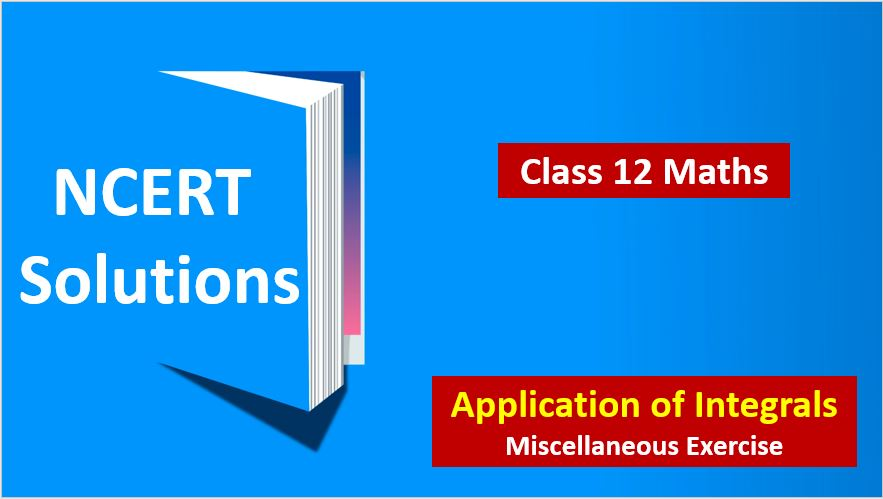# NCERT Solutions for Class 12 Maths Application of Integrals Miscellaneous Exercise

Hi Students, Welcome to Amans Maths Blogs (AMB). In this post, you will get the NCERT Solutions for Class 12 Maths Application of Integrals Miscellaneous Exercise.

CBSE Class 12th is an important school class in your life as you take some serious decision about your career. And out of all subjects, Maths is an important and core subjects. So CBSE NCERT Solutions for Class 12th Maths is major role in your exam preparation as it has detailed chapter wise solutions for all exercise. This NCERT Solutions can be downloaded in PDF file. The downloading link is given at last.

NCERT Solutions for class 12 is highly recommended by the experienced teacher for students who are going to appear in CBSE Class 12 and JEE Mains and Advanced and NEET level exams. Here You will get NCERT Solutions for Class 12 Maths Application of Integrals Miscellaneous Exercise of all questions given in NCERT textbooks of class 12 in details with step by step process.

NCERT Solutions for Class 12 Maths are not only the solutions of Maths exercise but it builds your foundation of other important subjects. Getting knowledge of depth concept of CBSE Class 12th Maths like Algebra, Calculus, Trigonometry, Coordinate Geometry help you to understand the concept of Physics and Physical Chemistry.

As we know that all the schools affiliated from CBSE follow the NCERT books for all subjects. You can check the CBSE NCERT Syllabus. Thus, NCERT Solutions helps the students to solve the exercise questions as given in NCERT Books

The PDF books of NCERT Solutions for Class 12 are the first step towards the learning and understanding the each sections of Maths, Physics, Chemistry, Biology as it all help in engineering medical entrance exams. To solve it, you just need to click on download links of NCERT solutions for class 12.

## NCERT Solutions for Class 12 Maths Application of Integrals Miscellaneous Exercise

NCERT Solutions Class 12 Math Application of Integrals Miscellaneous Exercise Ques No 1

Find the area under the given curves and given lines:
(i) y = x2, x = 1, x = 2 and x-axis

NCERT Solutions: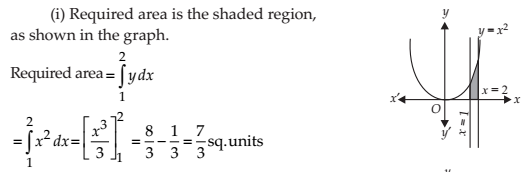(ii) y = x4, x = 1, x = 5 and x-axis

NCERT Solutions:NCERT Solutions Class 12 Math Application of Integrals Miscellaneous Exercise Ques No 2

Find the area between the curves y = x and y = x2.

NCERT Solutions:NCERT Solutions Class 12 Math Application of Integrals Miscellaneous Exercise Ques No 3

Find the area of the region lying in the first quadrant and bounded by y = 4x2, x = 0, y = 1 and y = 4.

NCERT Solutions:NCERT Solutions Class 12 Math Application of Integrals Miscellaneous Exercise Ques No 4

Sketch the graph of y = x + 3 and evaluate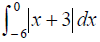.

NCERT Solutions: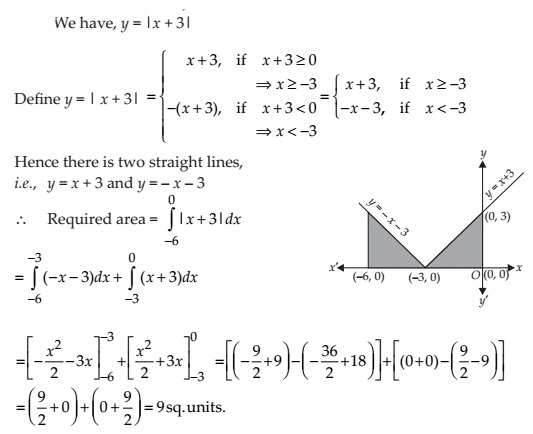NCERT Solutions Class 12 Math Application of Integrals Miscellaneous Exercise Ques No 5

Find the area bounded by the curve y = sin x between x = 0 and x = 2π.

NCERT Solutions: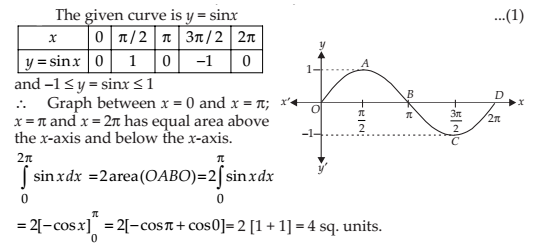NCERT Solutions Class 12 Math Application of Integrals Miscellaneous Exercise Ques No 6

Find the area enclosed between the parabola y2 = 4ax and the line y = mx.

NCERT Solutions:NCERT Solutions Class 12 Math Application of Integrals Miscellaneous Exercise Ques No 7

Find the area enclosed by the parabola 4y = 3x2 and the line 2y = 3x + 12.

NCERT Solutions: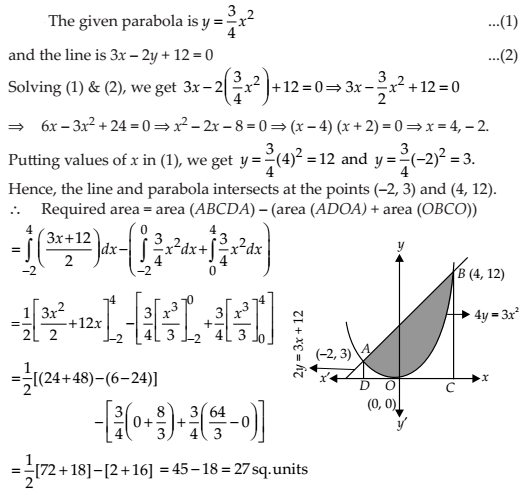NCERT Solutions Class 12 Math Application of Integrals Miscellaneous Exercise Ques No 8

Find the area of the smaller region bounded by the ellipse x2/9 + y2/4 = 1 and x/3 + y/2 = 1.

NCERT Solutions: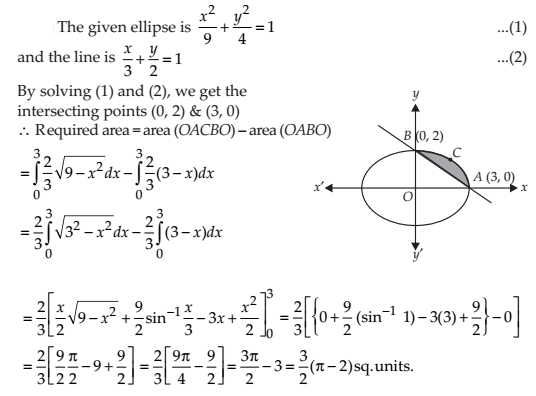NCERT Solutions Class 12 Math Application of Integrals Miscellaneous Exercise Ques No 9

Find the area of the smaller region bounded by the ellipse x2/a + y2/b = 1 and x/a + y/b = 1.

NCERT Solutions:NCERT Solutions Class 12 Math Application of Integrals Miscellaneous Exercise Ques No 10

Find the area of the region enclosed by the parabola x2 = y, the line y = x + 2 and the x-axis.

NCERT Solutions:NCERT Solutions Class 12 Math Application of Integrals Miscellaneous Exercise Ques No 11

Using the method of integration find the area bounded by the curve |x| + |y| = 1 .

NCERT Solutions: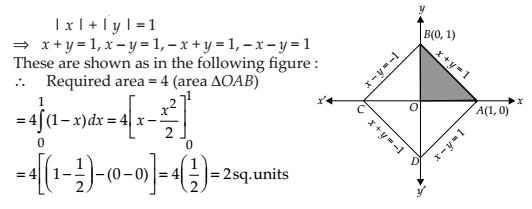NCERT Solutions Class 12 Math Application of Integrals Miscellaneous Exercise Ques No 12

Find the area bounded by curves {(x, y) : y ≥ x2 and y = | x |}.

NCERT Solutions: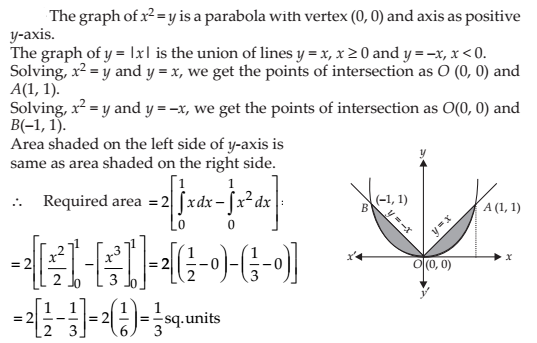NCERT Solutions Class 12 Math Application of Integrals Miscellaneous Exercise Ques No 13

Using the method of integration find the area of the triangle ABC, coordinates of whose vertices are A(2, 0), B (4, 5) and C (6, 3).

NCERT Solutions: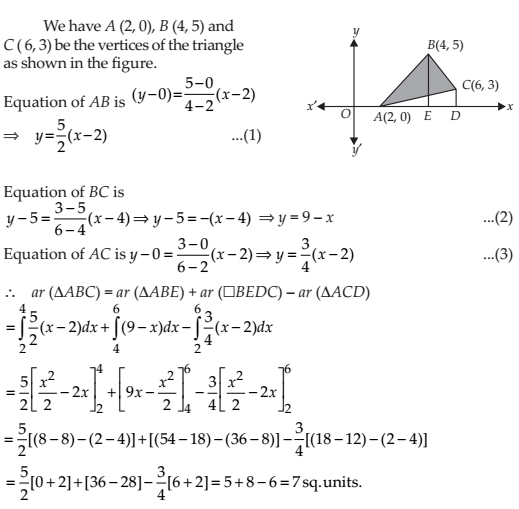NCERT Solutions Class 12 Math Application of Integrals Miscellaneous Exercise Ques No 14

Using the method of integration find the area of the region bounded by lines: 2x + y = 4, 3x – 2y = 6 and x – 3y + 5 = 0

NCERT Solutions:NCERT Solutions Class 12 Math Application of Integrals Miscellaneous Exercise Ques No 15

Find the area of the region {(x, y) : y2 ≤ 4x, 4x2 + 4y2 ≤ 9}.

NCERT Solutions: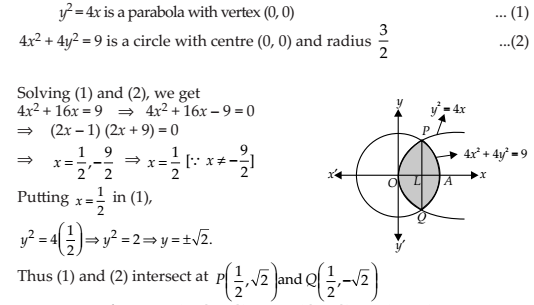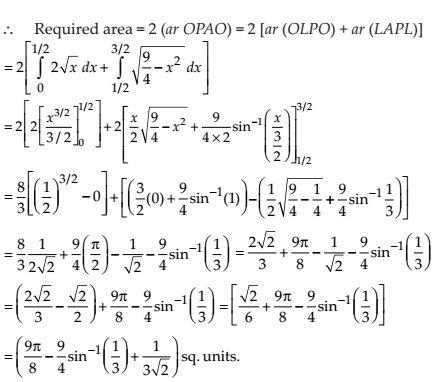Choose the correct answer in the following Exercises from 16 to 20.

NCERT Solutions Class 12 Math Application of Integrals Miscellaneous Exercise Ques No 16

Area bounded by the curve y = x3, the x-axis and the ordinates x = – 2 and x = 1 is

(A) – 9

(B) – 15/4

(C) 15/4

(D) 17/4

NCERT Solutions: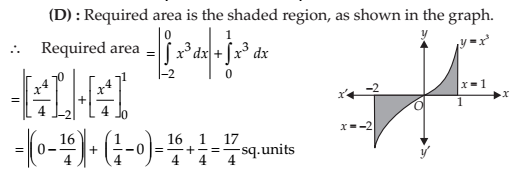NCERT Solutions Class 12 Math Application of Integrals Miscellaneous Exercise Ques No 17

The area bounded by the curve y = x | x | , x-axis and the ordinates x = – 1 and x = 1 is given by

(A) 0

(B) 1/3

(C) 2/3

(D) 4/3

NCERT Solutions:NCERT Solutions Class 12 Math Application of Integrals Miscellaneous Exercise Ques No 18

The area of the circle x2 + y2 = 16 exterior to the parabola y2 = 6x isNCERT Solutions: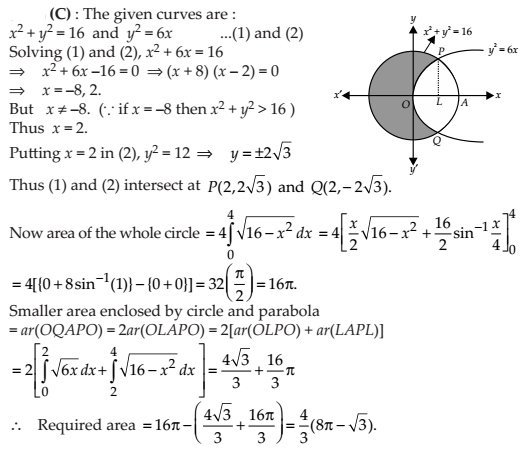NCERT Solutions Class 12 Math Application of Integrals Miscellaneous Exercise Ques No 19

The area bounded by the y-axis, y = cos x and y = sin x when 0 ≤ x ≤ π/2 is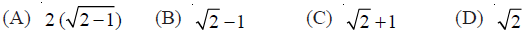NCERT Solutions:error: Content is protected !!